## NUMBERS AND OPERATIONS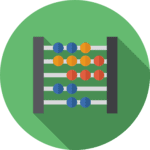The addition is the mathematical composition operation that consists of combining, joining or adding two or more numbers to obtain a final or total quantity.

In the additive expression, four fundamental properties are always satisfied:

1) Commutative property:

A modification in the order of its elements (addends) will not modify the result; for example: 3 + 2 = 2 + 3

2) Associative property:

When three or more numbers are added, the order of the addends does not influence the result; for example: 4 + (2 + 1) = (4 + 2) + 1

3) Property of the neutral element (or identity element):

Any number added to the number zero (0) will result in the same number; for example: 5 + 0 = 5

4) Distributive property (combines multiplication and addition):

The result of the sum of two numbers (A and B) multiplied by a third number (C) will be equivalent to the sum of their products (A x C + B x C); for example: 2 x (4 + 3) = 2 x 4 + 2 x 3

#### Properly place the terms of the addition in natural numbers up to 99,999

What are the "addends" and the "result" in a sum?

In the next lesson we will explain to you which are the terms or elements of any sum (addends and result) as well as the meaning of their mathematical symbols "+" and "= ".

- Addends: They represent each of the figures or numbers that we are going to add, that is, they correspond to the sets that we will join, combine and / or group.

- Result or Sum: Symbolizes the figure or number resulting from the sum. It corresponds to the unique set formed by joining, combining and / or grouping the quantities of the addends.How to build the algorithm of a vertical sum?

Next you will learn to construct vertical sums by placing the symbols "+" and "=" and each of their terms (addends and result) in the corresponding place, practicing with the different printable educational worksheets that we have developed for whole numbers from 0 to 100,000.

Remember, with the 4-digit and 5-digit additions you must always align, according to their place value, the ones (1s), the tens (10s), the hundreds (100s), the thousands (1,000s) and the ten thousands (10,000s) before solving any addition operations.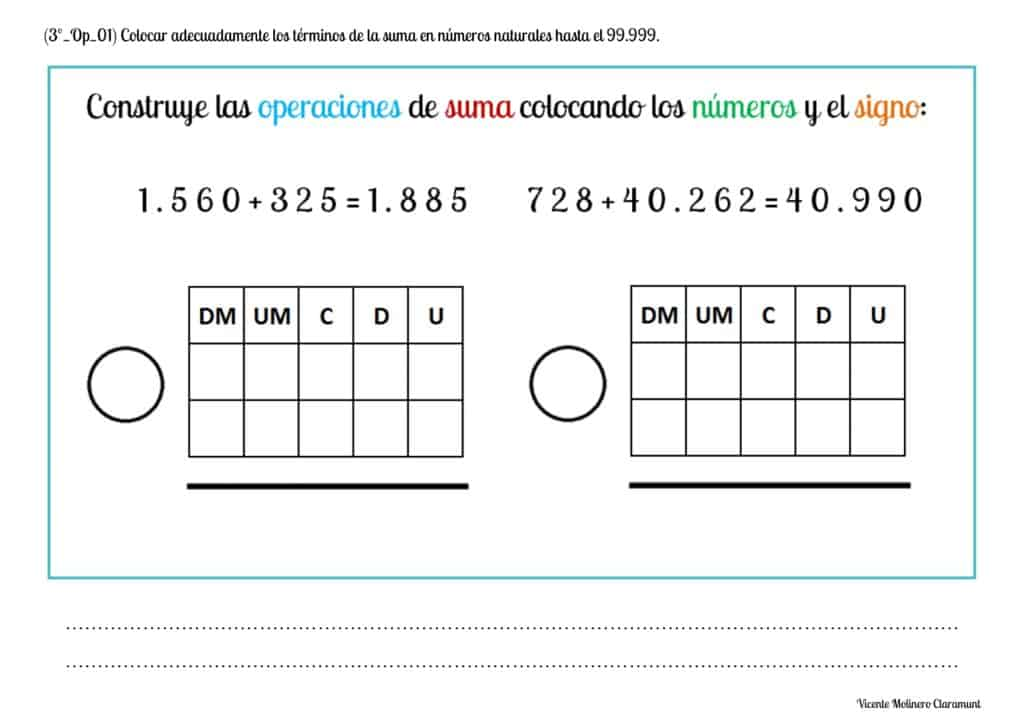#### Solve sums of two addends vertically without regrouping with natural numbers up to 99,999

How to add 4-digit and 5-digit numbers without carrying?

We present a solved example as well as some printable PDF activities accompanied by their explanation so that you can easily learn how to add in vertical and without regrouping four and five-digit numbers between 0 and the 100,000.

SPANISHENGLISH#### Solve sums of two addends vertically with regrouping with natural numbers up to 99,999

How to add 4-digit and 5-digit numbers with carrying?

Practice the 4 and 5-digits addition with regrouping with the whole numbers from 0 to 100,000 from our printable worksheets and become a mathematical expert.

If you need a little preparation, watch our video lessons where we provide you with some solved examples following the vertical resolution method by columns.

SPANISHENGLISH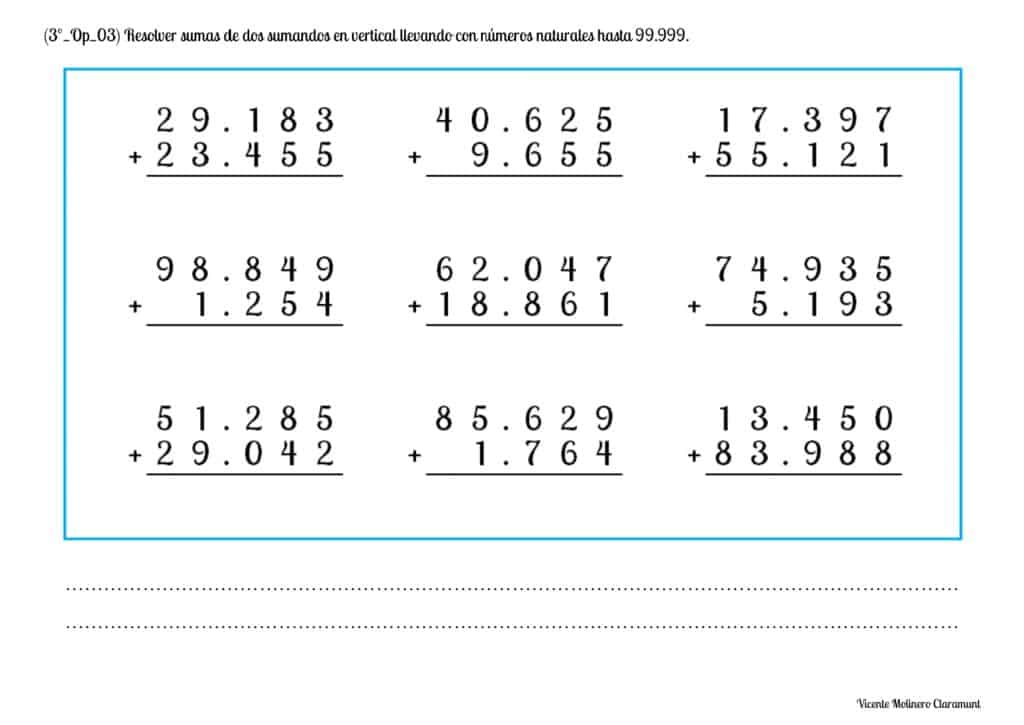We also provide you with a set of interactive games so that you can practice four and five-digit sums with carry from the personal computer (or desktop computer), the tablet and / or the mobile phone:

#### Solve sums of three addends vertically without regrouping with whole numbers up to 99,999

How to add 3 or more addends of up to 5 figures without carrying?

We explain step by step how to perform the calculations and how to find the result easily in the sums with three addends without carrying up to 5 digits.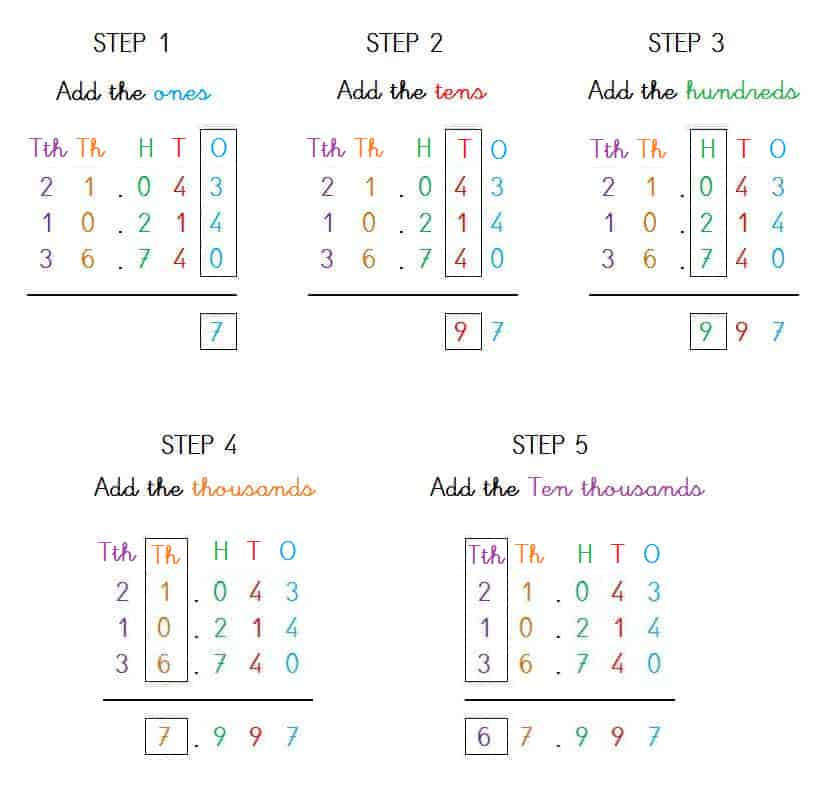#### Solve sums of three addends vertically with regrouping with whole numbers up to 99,999

How to add 3 or more addends of up to 5 figures with carrying?

Practice the addition of three addends with four and five digits and carried from our printable worksheets and with the natural numbers from 0 to 99,999.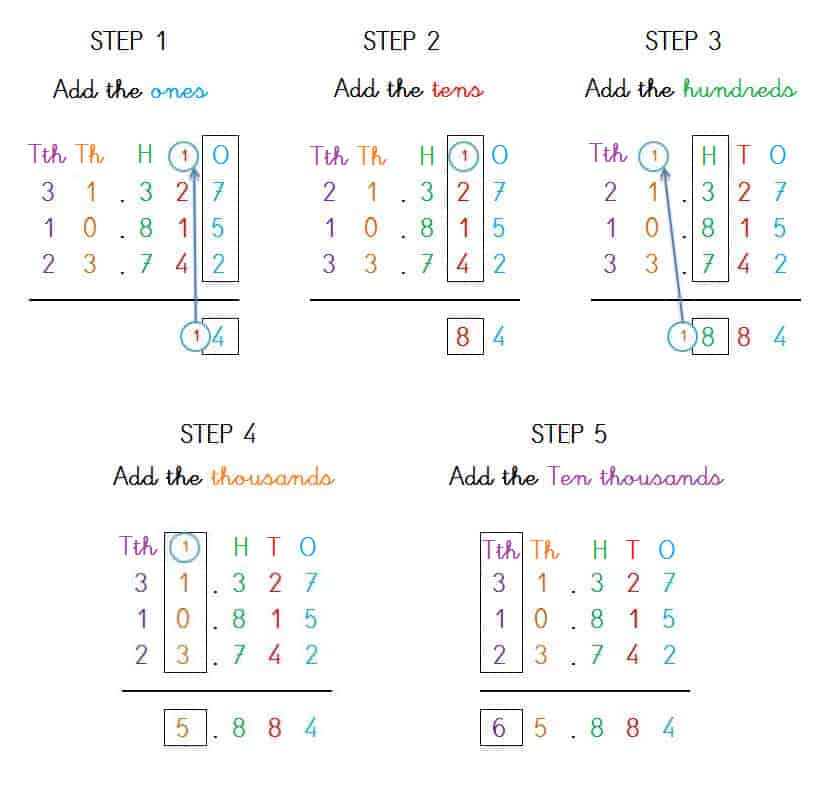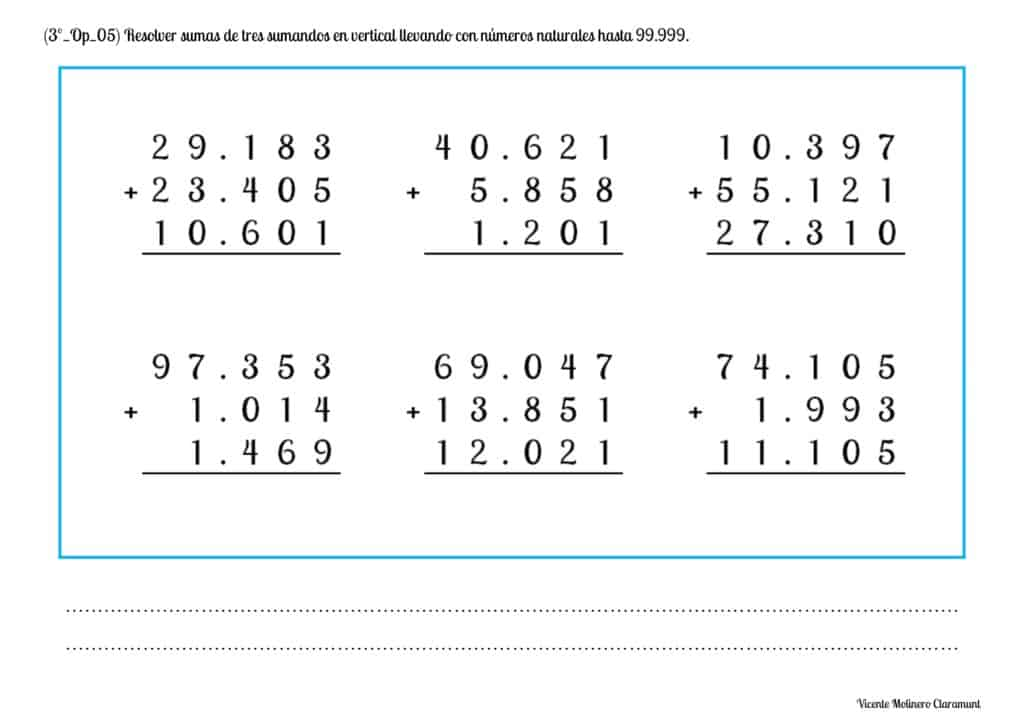#### Mentally solve simple addition facts with natural numbers up to 99,999

Show your logical-mathematical skills by solving our exercises with hidden or missing whole numbers and using mental calculation (also known as "mental math").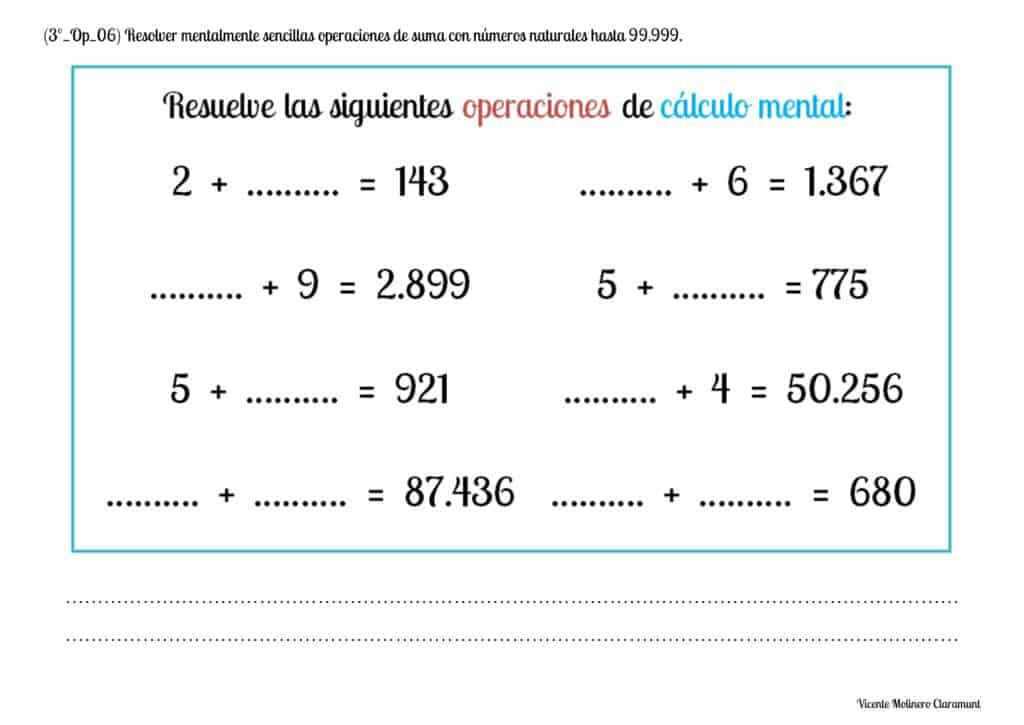#### Solve addition word problems without regrouping with natural numbers up to 99,999

We have made some exercises so that you can practice solving addition word problems no carrying with the numbers from 0 to 100,000.

To make it easier for you, we provide you a step-by-step explanation through a video tutorial with 4 and 5-digits adding word problems without regrouping.

SPANISHENGLISH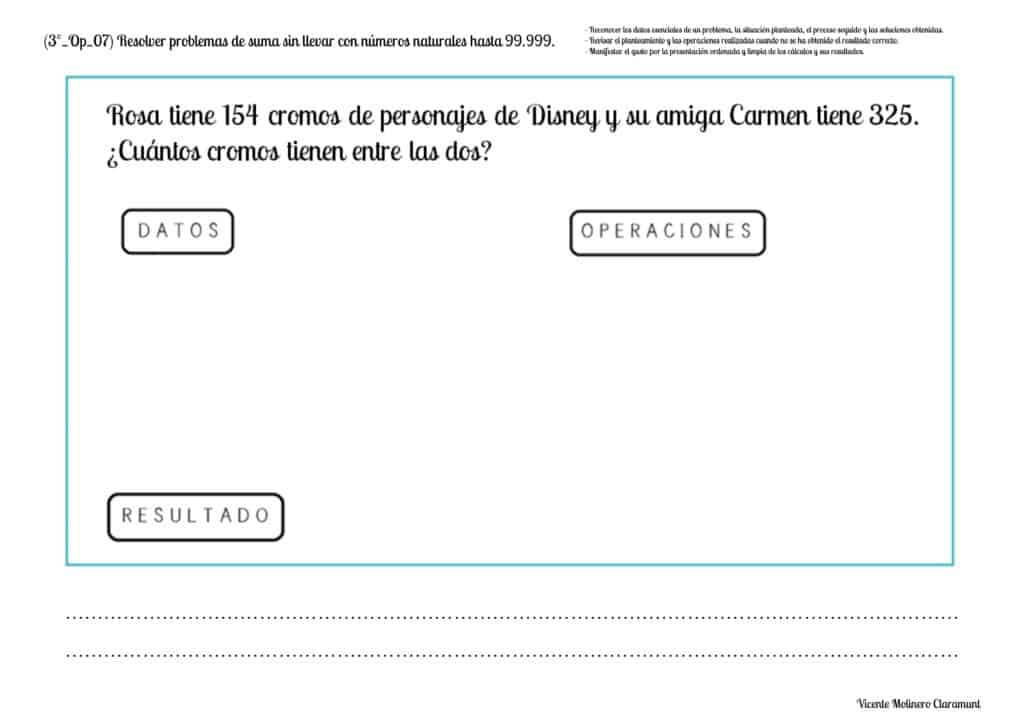#### Solve addition word problems with regrouping with whole numbers up to 99,999

Show that you are a math genius by solving each of our arithmetic problems using the basic operation of addition with carryover from 0 to 100,000.

If you need a little preparation, watch our video lessons where we provide you with some solved examples using the vertical column method.

SPANISHENGLISH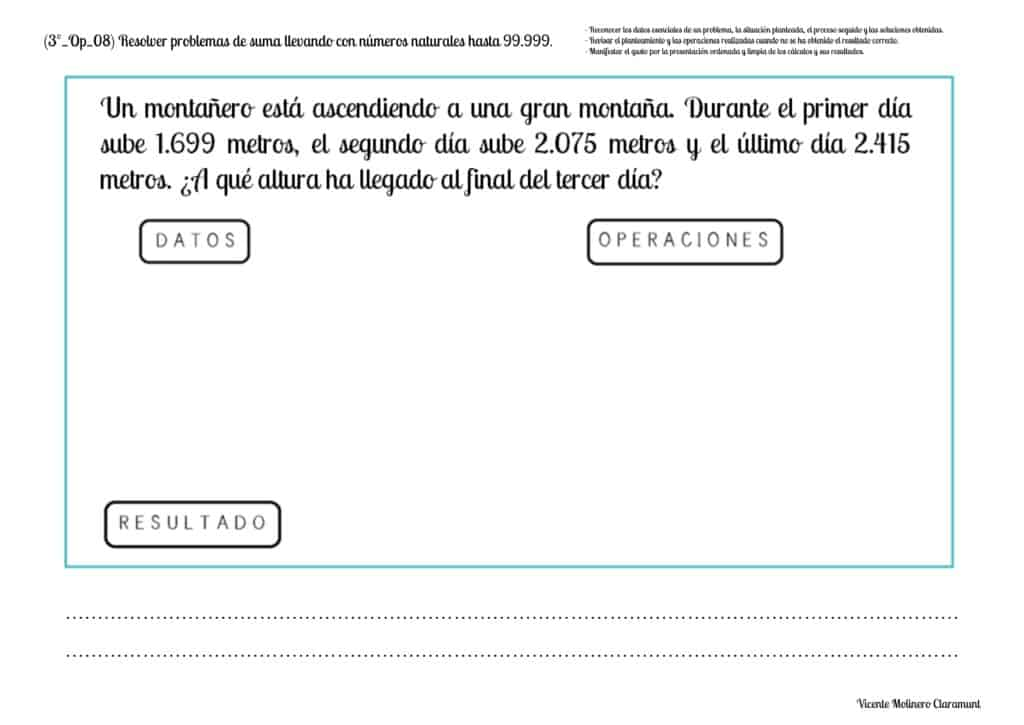#### Apply the commutative property of addition with natural numbers up to 99,999

Do you know what the Commutative Property of Addition is?

The Commutative Property of Addition is that universal mathematical law that states that "even if we change the order in which the addends are added, the result is always the same".

SPANISHENGLISH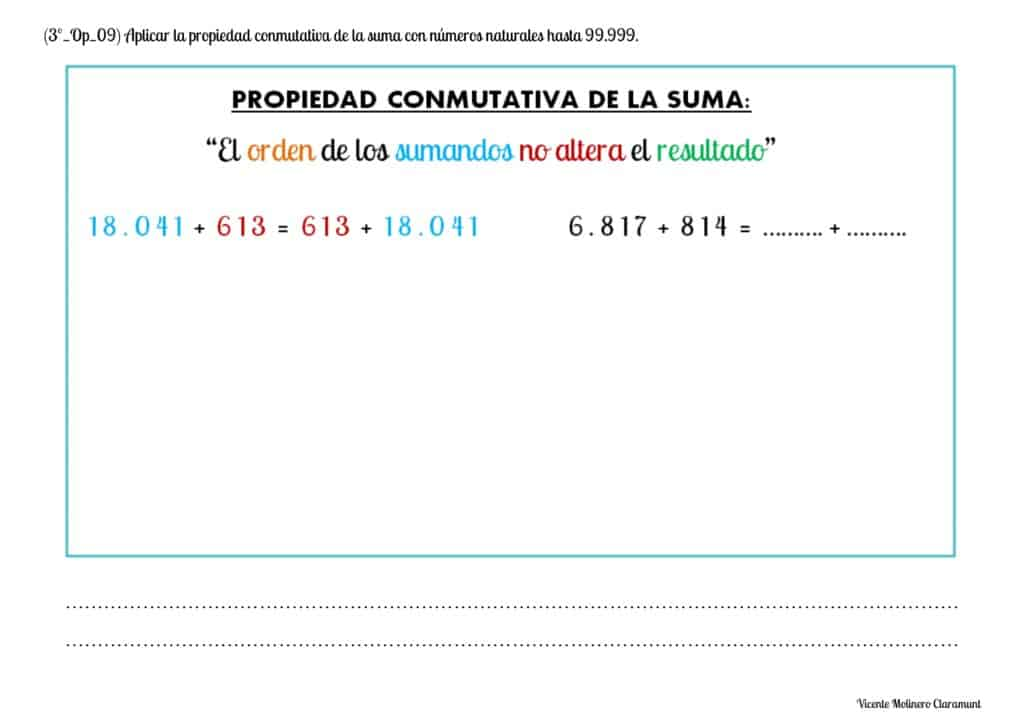#### Apply the associative property of addition with natural numbers up to 99,999

Do you know what the Associative Property of the Addition is?

The Associative Property of addition is that universal mathematical law that tells us that "if we add three or more numbers, the result will always be the same regardless of the order in which we group the addends".

SPANISHENGLISH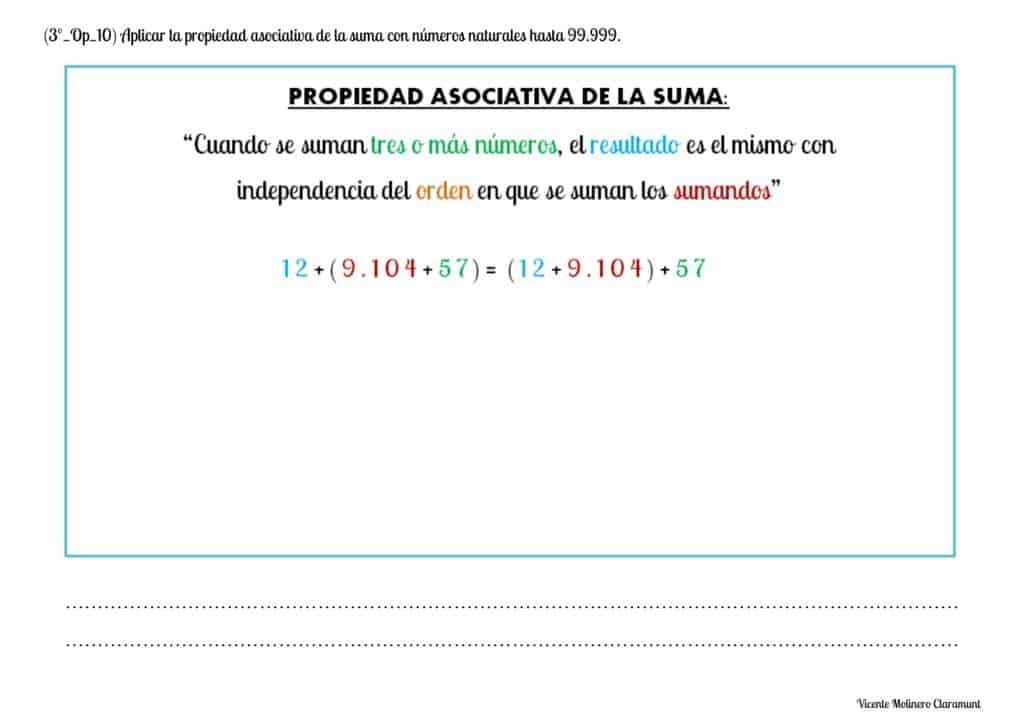Select more exercises, worksheets and activities of Mathematics for each of the 4 learning blocks of the Educational Curriculum ("Numbers and Operations", "Quantities and Measurements", "Geometric and Spatial Reasoning" and "Data Analysis and Probability") aimed at improving the logical-mathematical competencies and skills that are developed throughout "Grade 3" or "Year 4":